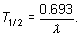# Physics: Nuclear and Atomic Flashcards Preview

## MCAT > Physics: Nuclear and Atomic > Flashcards

Flashcards in Physics: Nuclear and Atomic Deck (8)
1
Q

mass defect

A

when a common chemical bond is formed, some of the mass is converted into energy and realeased

Consequently, the mass of the nucleus formed is less than the sum of the masses of the constituent nucleons.

Mass defect= mnucleus - (Zmproton + Nmneutron)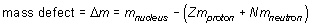2
Q

Which atom has the having the highest binding-energy per nucleon?

A

Iron.

General Trend: intermediate-sized nuclei are more stable than large or small nuclei.

3
Q

Binding Energy

A

mass defect is converted into the binding energy of the nucleus

BE = (Δm)c2

4
Q

alpha decay

A

After an alpha decay, the mass number of the daughter nucleus is always four less than the mass number of the parent nucleus, and the atomic number of the daughter nucleus is always two less than the atomic number of the parent nucleus.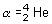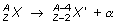5
Q

beta-decay (2 types)

A

beta-minus decay: the daughter nucleus has an atomic number one higher than the parent nucleus,

beta-plus decay: the daughter nucleus has an atomic number that is one less than the atomic number of the parent nucleus.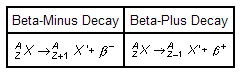6
Q

Gamma decay

A
• The decaying isotope doesn't change identity during a gamma decay.
• emission of gamma particles, which are high-energy photons.

• The emission of these high-energy photons means that the daughter nucleus has less energy than the parent nucleus. We say that the parent nucleus (X*) is an excited state, a state with a higher energy level, than the daughter nucleus (X).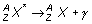7
Q

decay constant

A

If Nis the initial population of a certain sample of radioactive nuclei, then the population at time t satisfies: N=N0e-λt

As t increases, the value of N drops. The constant λ is called the decay constant; it characterizes the rate at which the population of nuclei decreases.

Plotting:

• lnN = ln N0 - λt
•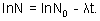•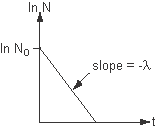• The y-intercept is the natural log of the initial population, and the slope of the line is the negative of the decay constant.
8
Q

half-life

A
• the average time it takes for half of the sample to decay
• (The greater the sample size, the more likely it is that exactly half the population will decay in time   T1/2.)
• T1/2 = 0.693/ λ